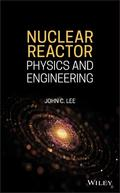John Wiley & Sons Nuclear ReactorAn introductory text for broad areas of nuclear reactor physics Nuclear Reactor Physics and Enginee.. Product #: 978-1-119-58232-8 Regular price: \$116.82 \$116.82 Auf Lager

# Nuclear Reactor

## Physics and Engineering

Wiley - IEEE1. Auflage April 2020
656 Seiten, Hardcover
Wiley & Sons Ltd

ISBN: 978-1-119-58232-8
John Wiley & Sons

Jetzt kaufen

### Preis: 125,00 €

Preis inkl. MwSt, zzgl. Versand

An introductory text for broad areas of nuclear reactor physics

Nuclear Reactor Physics and Engineering offers information on analysis, design, control, and operation of nuclear reactors. The author--a noted expert on the topic--explores the fundamentals and presents the mathematical formulations that are grounded in differential equations and linear algebra.

The book puts the focus on the use of neutron diffusion theory for the development of techniques for lattice physics and global reactor system analysis. The author also includes recent developments in numerical algorithms, including the Krylov subspace method, and the MATLAB software, including the Simulink toolbox, for efficient studies of steady-state and transient reactor configurations. In addition, nuclear fuel cycle and associated economics analysis are presented, together with the application of modern control theory to reactor operation. This important book:
* Provides a comprehensive introduction to the fundamental concepts of nuclear reactor physics and engineering
* Contains information on nuclear reactor kinetics and reactor design analysis
* Presents illustrative examples to enhance understanding
* Offers self-contained derivation of uid conservation equations

Written for undergraduate and graduate students in nuclear engineering and practicing engineers, Nuclear Reactor Physics and Engineering covers the fundamental concepts and tools of nuclear reactor physics and analysis.

Preface xiv

List of Tables xviii

List of Figures xix

1 Nuclear Power Plants 1

2 Neutronnucleus Reaction and Neutron Cross Section 27

3 Neutron Flux, Reaction Rate, and Effective Cross Section 59

4 Derivation of the Neutron Diffusion Equation 77

5 Applications of the One-Group Neutron Diffusion Equation 99

6 Numerical Solution of the Neutron Diffusion Equation 131

7 Applications of the Two-Group Neutron Diffusion Equation 165

8 Nuclear Reactor Kinetics 181

9 Fast Neutron Spectrum Calculation 219

10 Perturbation Theory and Adjoint Flux 255

11 Lattice Physics Analysis of Heterogeneous Cores 273

12 Nuclear Fuel Cycle Analysis and Management 323

13 Thermal-Hydraulic Analysis of Reactor Systems 373

14 Power Coefficients of Reactivity 455

15 Nuclear Energy Economics 471

16 Space-Time Kinetics and Reactor Control 489

17 Elements of Neutron Transport Theory 543

Appendix A: Key Physical Constants 575

Appendix B: Comparison of Major Reactor Types 577

Appendix C: Special Mathematical Functions 581

Appendix D: Integral Transforms 591

Appendix E: Calculus of Variation for Optimal Control Formulation 595

Appendix F: Kalman Filter Algorithm 603